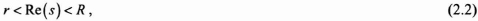## 2.2. The z-transform

### 2.2.1. Representations and summaries

With analog systems, the Laplace transform Xs(s) related to a continuous function x(t), is a function of a complex variable s and is represented by:Chapter written by Mohamed NAJIM and Eric GRIVEL.

This variable exists when the real part of the complex variable s satisfies the relation:with r = −∞ and R = +∞, r and R potentially characterizing the existence of limits of Xs, (s).

The Laplace transform helps resolve the linear differential equations to constant coefficients by transforming them into algebraic products.

Similarly, we introduce the z-transform when studying discrete-time signals.

Let {x(k)} be a real sequence. The bilaterial or two-sided z-transform Xz(z) of the sequence {x(k)} is represented as follows:where z is a complex variable. The relation (2.3) is sometimes called the direct z-transform since this makes it possible to transform the time-domain signal {x(k)} into a representation in the complex-plane.

The z-transform only exists for the values of z that enable the series to converge; that is, for the value of z so that Xz(z) has a finite value. The set of all values of z satisfying these properties is ...

Get Digital Filters Design for Signal and Image Processing now with the O’Reilly learning platform.

O’Reilly members experience books, live events, courses curated by job role, and more from O’Reilly and nearly 200 top publishers.